# Author: Ivana Nikoloska

Whilst the true impact of quantum computers is anybody’s guess, there seems to be some consensus on the advantages offered by near-term devices in modeling more complex probability distributions. These distributions can be used to model complex particle interactions, e.g., in quantum chemistry, or, as we will see next, to train principled machine learning models – in this case, binary Bayesian neural networks – and enable fast adaptation to new learning tasks from few training examples.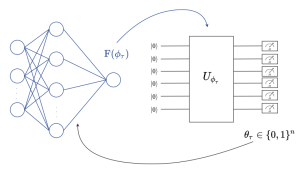Fig. 1. (left) A binary Bayesian neural network, i.e., a neural network with stochastic binary weights, is trained to carry out a learning task. (right) The probability distribution of the binary weights of the neural network is modelled by a Born machine, i.e., by a parametric quantum circuit (PQC), leveraging the PQC’s capacity to model complex distributions .

## Setting

In our latest work, accepted for presentation at the IEEE MLSP, we are interested in training Bayesian binary neural networks, i.e., classical neural networks with stochastic binary weights, in a sample-efficient manner by means of meta-learning, as illustrated in Fig. 1. The key idea of this work is to model the distribution of the binary weights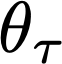via a Born machine, i.e., via a probabilistic parametric quantum circuit (PQC), due to the capacity of PQCs to efficiently implement complex probability distributions -. We propose a novel method that integrates meta-learning with the gradient-based optimization of quantum Born machines , with the aim of speeding up adaptation to new learning tasks from few examples.

## Born Machines

A Born machine produces random binary strings, wheredenotes the total number of model parameters, by measuring the output of a PQCdefined by parameters.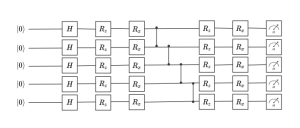Fig. 2. Hardware-efficient ansatz for a Born machine. All qubits are initialized in the ground state. The rotations are parametrized by the entries of the variational vector.

As illustrated in Fig. 2, the PQC takes the initial stateof n qubits as an input, and operates on it via a sequence of unitary gates described by a unitary matrix. This operation outputs the final quantum state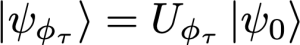which is measured in the computational basis to produce a random binary string. Note that each basis vector of the computational basis corresponds to one of all the possible 2^n patterns of model parameters.

The PQC can be implemented using a hardware-efficient ansatz , in which a layer of one-qubit unitary gates, parametrized by vector, is followed by a layer of fixed, entangling, two-qubit gates. This pattern can be repeated any number of times, building a progressively deeper circuit. Another option is using the mean-field ansatz that does not use entangling gates, and only relies on one-qubit gates.

By Born’s rule (hence the name of the circuit), the probability distribution of the output model parameter vectoris given by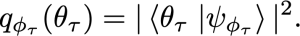Importantly, Born machines only provide samples, while the actual distribution above can only be estimated by averaging multiple measurements of the PQC’s outputs. Therefore, Born machines model implicit distributions, and only define a stochastic procedure that directly generates samples.

## Some Results

Fig. 3 illustrate the results in terms of the prediction root mean squared error (RMSE) as a function of the number of meta-training iterations. By comparison with conventional per-task learning, the figure illustrates the capacity of both joint learning and meta-learning to transfer knowledge from the meta-training to the meta-test task, with hardware-efficient (HE) and mean-field (MF) quantum meta-learning clearly outperforming joint learning. For example, HE meta-learning requires around 150 meta-training iterations to achieve the same RMSE ideal per-task training, whilst joint-learning requires more than 200 to achieve comparable performance. The HE ansatz performs best, due to the use of entangling unitaries; however, the MF ansatz approaches the minimal RMSE after 230 iterations. The classical solution based on MF Bernoulli does not achieve lower RMSE than the quantum-aided meta-learning schemes, even with joint learning.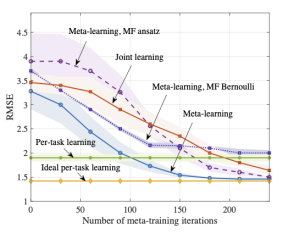Fig. 3. Average RMSE for a new, meta-test, task as a function of the number of meta-training iterations. The results are averaged over 5 independent trials.

Please see the paper for a more detailed exposition, available here.

## References

 Arute, F., Arya, K., Babbush, R., Bacon, D., Bardin, J.C., Barends, R., Biswas, R., Boixo, S., Brandao, F.G., Buell, D.A., et al.: Quantum supremacy using a programmable superconducting processor. Nature 574(7779), 505–510 (2019)
 Kandala, A., Mezzacapo, A., Temme, K., Takita, M., Brink, M., Chow, J.M., Gambetta, J.M.: Hardware-efficient variational quantum eigensolver for small molecules and quantum magnets. Nature 549(7671), 242–246 (2017)
 Liu, J.G., Wang, L.: Differentiable learning of quantum circuit Born machines. Physical Review A 98(6), 062324 (2018)
 Sweke, R., Seifert, J.P., Hangleiter, D., Eisert, J.: On the quantum versus classical learnability of discrete distributions. Quantum 5, 417 (2021)

In 1959 Richard Feynman gave an after-dinner talk at an American Physics Society meeting in Pasadena entitled “There’s Plenty of Room at the Bottom”,  crediting Edward Fredkin for inspiration.  In his talk, the transcription of which would later become a landmark paper in quantum computation and simulation , he takes some existing ideas — computation is a physical process, perhaps even a quantum mechanical one — and makes a particularly famous statement:

”I’m not happy with all the analyses that go with just classical theory, because Nature isn’t classical, dammit, and if you want to make a simulation of Nature, you’d better make it quantum mechanical, and by golly it’s a wonderful problem!”

But how can we simulate the quantum mechanical nature of Nature? This new kind of machine would become the quantum computer, and from then on, quantum computing has been on a journey with many ups and downs. Nowadays, excitement seems to be in the air again as quantum machine learning, a hybrid research discipline that combines machine learning and quantum computing, has emerged as a potential practical use of quantum hardware. Generally, quantum machine learning methods apply classical optimization routines to select parameters that define the operation of a quantum circuit. Alternative approaches, which may be more promising in the short term, involve hybrid quantum-classical models, where classical computation, e.g., for feature extraction, is combined with quantum parametric circuits .

## Our Work

In our latest work, published in the IEEE Signal Processing Letters, we focus on the hybrid classical-quantum two-layer architecture illustrated in Fig. 1.Fig. 1. In the studied hybrid classical-quantum classifier, a quantum hidden layer, fed via amplitude encoding and consisting of quantum generalized linear models (QGLMs), is followed by a classical combining output layer with a single classical GLM (CGLM) neuron. All weights and activations are binary.

In it, a first layer of quantum generalized linear models (QGLMs) is followed by a second classical combining layer. The input to the first, hidden, layer is obtained via amplitude encoding (see, e.g., ). Several implementations of QGLM neurons have been proposed in the literature using different quantum circuits. Given a binary input sampleand an N-dimensional vectorof binary weights, the main goal of these circuits is to produce a stochastic binary output with probabilities which are a function of the inner product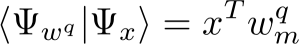between the input stateand the amplitude-encoded binary weight vectorDifferent solutions, along with the resulting QGLM neuron’s response functions are given in the paper. For this hybrid model, we introduced a stochastic variational optimization (SVO) approach  that enables the joint training of quantum and classical layers via stochastic gradient descent. The proposed SVO-based training strategy operates in a relaxed continuous space of variational classical parameters.

## Some Results

We show the classification accuracy, which is defined as the ratio of the number of accurate predictions over the total number of predictions made by the model, in Fig. 2 as a function of the training iterations.Fig. 2. Classification accuracy as a function of the training iteration for the benchmark sign-flips scheme  and the proposed SVO-based procedure for the BAS data set. The results are averaged over 5 independent trials.

The proposed SVO scheme is seen to achieve high classification accuracy for all of the considered response functions. In particular, the QGLM using the Quadratic (Q) response function yields fastest convergence and achieves the best performance. Due to the additional bias terms resulting from the swap test routine, the QGLMs relying on the Biased quadratic (BQ) and Biased centered quadratic (BCQ) response functions are slower to learn, but ultimately converge after around 3000 training iterations.

Please see the paper for a more extensive presentation, available here

Code, alongside a tutorial, are available here

## References

 R. P. Feynman et al., “Simulating physics with computers,” Int. j. Theor. phys, vol. 21, no. 6/7, 1982.
 A. Mari, T. R. Bromley, J. Izaac, M. Schuld, and N. Killoran, “Transfer learning in hybrid classical-quantum neural networks,” Quantum, vol. 4, p. 340, 2020.
 M. Schuld and F. Petruccione, Machine Learning with Quantum Computers. Springer, 2021.
 T. Bird, J. Kunze, and D. Barber, “Stochastic variational optimization,” arXiv preprint arXiv:1809.04855, 2018.
 F. Tacchino, C. Macchiavello, D. Gerace, and D. Bajoni, “An artificial neuron implemented on an actual quantum processor,” npj Quantum Information, vol. 5, no. 1, pp. 1–8, 2019

### Problem

An essential property of any wireless channel is the fact that it is a shared medium, much like the air through which sound propagates is shared among the participants of a conversation. As a result, communication engineers must deal with the resulting interference,  which may substantially limit the reliability and the achievable rates in a wireless communication system. A proven remedy is to adapt the transmission power to current channel conditions, which was successfully addressed by the data-driven methodology introduced in  in which the power control policy is parametrized by a random edge graph neural network (REGNN).

In our recent work to be presented at SPAWC 2021, we focus on the higher-level problem of facilitating adaptation of the power control policy. We consider the case where the topology of the network varies across periods of operation of the system, with each period being in turn characterized by time-varying channel conditions. In order to facilitate fast adaptation of the power control policy — in terms of data and iteration requirements — we integrate meta-learning with REGNN training.

### Meta-learning Solution

Our meta-learning solution leverages channel state information (CSI) data from a number of previous periods to optimize an adaptation procedure that facilitates fast adaptation on a new topology to be encountered in a future period. We specifically adopt first-order meta-learning methods, namely first-order model agnostic meta-learning (FOMAML)  and REPTILE  that parametrize the adaptation procedure via its initialization within each period. While GNNs are known to be robust to changes in the topology, the proposed integration of meta-learning and REGNNs is shown to offer significant improvements in terms of sample and iteration efficiency.Fig 1. Sum rate as a function of the number of samples used for adaptation, for a network with dynamic size.

### Some Results

The achievable sum rate with respect to the number of CSI samples used for adaptation is illustrated in Fig. 1 for a network in which the number of transmitters and receivers changes in each period. Meta-learning, via both FOMAML and REPTILE, is seen to adapt quickly to the new topology, outperforming conventional REGNN, even when allowing for fine-tuning of the later. This significant improvement can be attributed to the variability of the topologies observed across periods in the considered scenario, which makes the joint training approach in  ineffective. That said, when the number of samples for adaptation is sufficiently large, conventional REGNN training as in  outperforms meta-learning, as the initialization obtained by meta-learning induces a more substantial bias than joint training due to the mismatch in the conditions assumed for the updates on meta-training and meta-testing tasks (i.e., the different number of samples used for meta-training and adaptation).

Please see the paper for more results and a more extensive analysis, which is available here

 M. Eisen and A. Ribeiro, “Optimal wireless resource allocation with random edge graph neural networks,”IEEE Transactionson Signal Processing, vol. 68, pp. 2977–2991, April, 2020.

 C. Finn, P. Abbeel, and S. Levine, “Model-agnostic meta-learning for fast adaptation of deep networks,” inProc. InternationalConference on Machine Learning (PMLR). Sydney, 6–11 August, 2017, pp. 1126–1135.

 A. Nichol, J. Achiam, and J. Schulman, “On first-order meta-learning algorithms,”arXiv preprint arXiv:1803.02999, 2018.